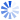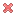# Online C Programming Test - C Programming Test 10Loading Test...

Instruction:

• Total number of questions : 20.
• Time alloted : 20 minutes.
• Each question carry 1 mark, no negative marks.
• DO NOT refresh the page.
• All the best :-).

1.

Point out the correct statements are correct about the program below?

``````#include<stdio.h>
int main()
{
char ch;
while(x=0;x<=255;x++)
printf("ASCII value of %d character %c\n", x, x);
return 0;
}
``````

A.
 The code generates an infinite loopB.
 The code prints all ASCII values and its charactersC.
 Error: x undeclared identifierD.
 Error: while statement missingYour Answer: Option (Not Answered)

Correct Answer: Option D

Explanation:

There are 2 errors in this program.
1. "Undefined symbol x" error. Here x is not defined in the program.
2. Here while() statement syntax error.

Learn more problems on : Control Instructions

Discuss about this problem : Discuss in Forum

2.

Which of the following is the correct usage of conditional operators used in C?

A.
 a>b ? c=30 : c=40;B.
 a>b ? c=30;C.
 max = a>b ? a>c?a:c:b>c?b:cD.
 return (a>b)?(a:b)Your Answer: Option (Not Answered)

Correct Answer: Option C

Explanation:

Option A: assignment statements are always return in paranthesis in the case of conditional operator. It should be a>b? (c=30):(c=40);

Option B: it is syntatically wrong.

Option D: syntatically wrong, it should be return(a>b ? a:b);

Option C: it uses nested conditional operator, this is logic for finding greatest number out of three numbers.

Learn more problems on : Expressions

Discuss about this problem : Discuss in Forum

3.

What will be the output of the program?

``````#include<stdio.h>
#include<math.h>
int main()
{
float n=1.54;
printf("%f, %f\n", ceil(n), floor(n));
return 0;
}
``````

A.
 2.000000, 1.000000B.
 1.500000, 1.500000C.
 1.550000, 2.000000D.
 1.000000, 2.000000Your Answer: Option (Not Answered)

Correct Answer: Option A

Explanation:

ceil(x) round up the given value. It finds the smallest integer not < x.
floor(x) round down the given value. It finds the smallest integer not > x.

printf("%f, %f\n", ceil(n), floor(n)); In this line ceil(1.54) round up the 1.54 to 2 and floor(1.54) round down the 1.54 to 1.

In the printf("%f, %f\n", ceil(n), floor(n)); statement, the format specifier "%f %f" tells output to be float value. Hence it prints 2.000000 and 1.000000.

Learn more problems on : Floating Point Issues

Discuss about this problem : Discuss in Forum

4.

What will be the output of the program?

``````#include<stdio.h>
int main()
{
float d=2.25;
printf("%e,", d);
printf("%f,", d);
printf("%g,", d);
printf("%lf", d);
return 0;
}
``````

A.
 2.2, 2.50, 2.50, 2.5B.
 2.2e, 2.25f, 2.00, 2.25C.
 2.250000e+000, 2.250000, 2.25, 2.250000D.
 ErrorYour Answer: Option (Not Answered)

Correct Answer: Option C

Explanation:

printf("%e,", d); Here '%e' specifies the "Scientific Notation" format. So, it prints the 2.25 as 2.250000e+000.

printf("%f,", d); Here '%f' specifies the "Decimal Floating Point" format. So, it prints the 2.25 as 2.250000.

printf("%g,", d); Here '%g' "Use the shorter of %e or %f". So, it prints the 2.25 as 2.25.

printf("%lf,", d); Here '%lf' specifies the "Long Double" format. So, it prints the 2.25 as 2.250000.

Learn more problems on : Floating Point Issues

Discuss about this problem : Discuss in Forum

5.

If a function contains two return statements successively, the compiler will generate warnings. Yes/No ?

A.
 YesB.
 NoYour Answer: Option (Not Answered)

Correct Answer: Option A

Explanation:

Yes. If a function contains two return statements successively, the compiler will generate "Unreachable code" warnings.

Example:

``````
#include<stdio.h>
int mul(int, int); /* Function prototype */

int main()
{
int a = 4, b = 3, c;
c = mul(a, b);
printf("c = %d\n", c);
return 0;
}
int mul(int a, int b)
{
return (a * b);
return (a - b); /* Warning: Unreachable code */
}
``````

Output:
c = 12

Learn more problems on : Functions

Discuss about this problem : Discuss in Forum

6.

Point out the error in the program

``````#include<stdio.h>

int main()
{
int i;
#if A
printf("Enter any number:");
scanf("%d", &i);
#elif B
printf("The number is odd");
return 0;
}
``````

A.
 Error: unexpected end of file because there is no matching #endifB.
 The number is oddC.
 Garbage valuesD.
 None of aboveYour Answer: Option (Not Answered)

Correct Answer: Option A

Explanation:

The conditional macro #if must have an #endif. In this program there is no #endif statement written.

Learn more problems on : C Preprocessor

Discuss about this problem : Discuss in Forum

7.

What will be the output of the program ?

``````#include<stdio.h>

int main()
{
float arr[] = {12.4, 2.3, 4.5, 6.7};
printf("%d\n", sizeof(arr)/sizeof(arr));
return 0;
}
``````

A.
 5B.
 4C.
 6D.
 7Your Answer: Option (Not Answered)

Correct Answer: Option B

Explanation:

The sizeof function return the given variable. Example: float a=10; sizeof(a) is 4 bytes

Step 1: float arr[] = {12.4, 2.3, 4.5, 6.7}; The variable arr is declared as an floating point array and it is initialized with the values.

Step 2: printf("%d\n", sizeof(arr)/sizeof(arr));

The variable arr has 4 elements. The size of the float variable is 4 bytes.

Hence 4 elements x 4 bytes = 16 bytes

sizeof(arr) is 4 bytes

Hence 16/4 is 4 bytes

Hence the output of the program is '4'.

Learn more problems on : Arrays

Discuss about this problem : Discuss in Forum

8.

Is there any difference int the following declarations?
int fun(int arr[]);
int fun(int arr);

A.
 YesB.
 NoYour Answer: Option (Not Answered)

Correct Answer: Option B

Explanation:

No, both the statements are same. It is the prototype for the function fun() that accepts one integer array as an parameter and returns an integer value.

Learn more problems on : Arrays

Discuss about this problem : Discuss in Forum

9.

What will be the output of the program (Turbo C in 16 bit platform DOS) ?

``````#include<stdio.h>
#include<string.h>

int main()
{
char *str1 = "India";
char *str2 = "BIX";
char *str3;
str3 = strcat(str1, str2);
printf("%s %s\n", str3, str1);
return 0;
}
``````

A.
 IndiaBIX IndiaB.
 IndiaBIX IndiaBIXC.
 India IndiaD.
 ErrorYour Answer: Option (Not Answered)

Correct Answer: Option B

Explanation:

It prints 'IndiaBIX IndiaBIX' in TurboC (in 16 bit platform).

It may cause a 'segmentation fault error' in GCC (32 bit platform).

Learn more problems on : Strings

Discuss about this problem : Discuss in Forum

10.

Which of the following statements correct about the below program?

``````#include<stdio.h>

int main()
{
union a
{
int i;
char ch;
};
union a u1 = {512};
union a u2 = {0, 2};
return 0;
}
``````
 1: u2 CANNOT be initialized as shown. 2: u1 can be initialized as shown. 3: To initialize char ch[] of u2 '.' operator should be used. 4: The code causes an error 'Declaration syntax error'

A.
 1, 2B.
 2, 3C.
 1, 2, 3D.
 1, 3, 4Your Answer: Option (Not Answered)

Correct Answer: Option C

Learn more problems on : Structures, Unions, Enums

Discuss about this problem : Discuss in Forum

11.

Does there exist any way to make the command-line arguments available to other functions without passing them as arguments to the function?

A.
 YesB.
 NoYour Answer: Option (Not Answered)

Correct Answer: Option A

Explanation:

Using the predefined variables _argc, _argv. This is a compiler dependent feature. It works in TC/TC++ but not in gcc and visual studio.

Learn more problems on : Command Line Arguments

Discuss about this problem : Discuss in Forum

12.

What will be the output of the program?

``````#include<stdio.h>
int get();

int main()
{
const int x = get();
printf("%d", x);
return 0;
}
int get()
{
return 20;
}
``````

A.
 Garbage valueB.
 ErrorC.
 20D.
 0Your Answer: Option (Not Answered)

Correct Answer: Option C

Explanation:

Step 1: int get(); This is the function prototype for the funtion get(), it tells the compiler returns an integer value and accept no parameters.

Step 2: const int x = get(); The constant variable x is declared as an integer data type and initialized with the value "20".

The function get() returns the value "20".

Step 3: printf("%d", x); It prints the value of the variable x.

Hence the output of the program is "20".

Learn more problems on : Const

Discuss about this problem : Discuss in Forum

13.

Point out the error in the program.

``````#include<stdio.h>
#include<stdlib.h>
int fun(const union employee *e);

union employee
{
char name;
int age;
float salary;
};
const union employee e1;

int main()
{
strcpy(e1.name, "A");
fun(&e1);
printf("%s %d %f", e1.name, e1.age, e1.salary);
return 0;
}
int fun(const union employee *e)
{
strcpy((*e).name, "B");
return 0;
}
``````

A.
 Error: RValue requiredB.
 Error: cannot convert parameter 1 from 'const char' to 'char *'C.
 Error: LValue required in strcpyD.
 No errorYour Answer: Option (Not Answered)

Correct Answer: Option B

Learn more problems on : Const

Discuss about this problem : Discuss in Forum

14.

What function should be used to free the memory allocated by calloc() ?

A.
 dealloc();B.
 malloc(variable_name, 0)C.
 free();D.
 memalloc(variable_name, 0)Your Answer: Option (Not Answered)

Correct Answer: Option C

Learn more problems on : Memory Allocation

Discuss about this problem : Discuss in Forum

15.

Point out the correct statement which correctly free the memory pointed to by 's' and 'p' in the following program?

``````#include<stdio.h>
#include<stdlib.h>

int main()
{
struct ex
{
int i;
float j;
char *s
};
struct ex *p;
p = (struct ex *)malloc(sizeof(struct ex));
p->s = (char*)malloc(20);
return 0;
}
``````

A.
 free(p); , free(p->s);B.
 free(p->s); , free(p);C.
 free(p->s);D.
 free(p);Your Answer: Option (Not Answered)

Correct Answer: Option B

Learn more problems on : Memory Allocation

Discuss about this problem : Discuss in Forum

16.

What do the following declaration signify?

``void *cmp();``

A.
 cmp is a pointer to an void type.B.
 cmp is a void type pointer variable.C.
 cmp is a function that return a void pointer.D.
 cmp function returns nothing.Your Answer: Option (Not Answered)

Correct Answer: Option C

Learn more problems on : Complicated Declarations

Discuss about this problem : Discuss in Forum

17.

What will be the output of the program?

``````#include<stdio.h>

int main()
{
char huge *near *far *ptr1;
char near *far *huge *ptr2;
char far *huge *near *ptr3;
printf("%d, %d, %d\n", sizeof(**ptr1), sizeof(ptr2), sizeof(*ptr3));
return 0;
}
``````

A.
 4, 4, 4B.
 2, 2, 2C.
 2, 8, 4D.
 2, 4, 8Your Answer: Option (Not Answered)

Correct Answer: Option A

Learn more problems on : Complicated Declarations

Discuss about this problem : Discuss in Forum

18.

Point out the error in the following program.

``````#include<stdio.h>
void display(int (*ff)());

int main()
{
int show();
int (*f)();
f = show;
display(f);
return 0;
}
void display(int (*ff)())
{
(*ff)();
}
int show()
{
printf("IndiaBIX");
}
``````

A.
 Error: invalid parameter in function display()B.
 Error: invalid function call f=show;C.
 No error and prints "IndiaBIX"D.
 No error and prints nothing.Your Answer: Option (Not Answered)

Correct Answer: Option C

Learn more problems on : Complicated Declarations

Discuss about this problem : Discuss in Forum

19.

We can modify the pointers "source" as well as "target".

A.
 TrueB.
 FalseYour Answer: Option (Not Answered)

Correct Answer: Option A

Learn more problems on : Complicated Declarations

Discuss about this problem : Discuss in Forum

20.

Will the program outputs "IndiaBIX.com"?

``````#include<stdio.h>
#include<string.h>

int main()
{
char str1[] = "IndiaBIX.com";
char str2;
strncpy(str2, str1, 8);
printf("%s", str2);
return 0;
}
``````

A.
 YesB.
 NoYour Answer: Option (Not Answered)

Correct Answer: Option B

Explanation:

No. It will print something like 'IndiaBIX(some garbage values here)' .

Because after copying the first 8 characters of source string into target string strncpy() doesn't terminate the target string with a '\0'. So it may print some garbage values along with IndiaBIX.

Learn more problems on : Library Functions

Discuss about this problem : Discuss in Forum

Submit your test now to view the Results and Statistics with answer explanation.

#### Current Affairs 2019

Interview Questions and Answers